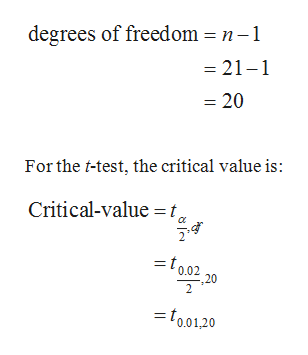# Find the critical value tc for the confidence level c=0.98 and sample size n=21

Question
392 views

Find the critical value tc for the confidence level c=0.98 and sample size n=21

check_circle

Step 1

The critical value tc for 98% confidence level with sample size 21:

The confidence level is 98%. That is, the level of significance is 1–0.98 = 0.02. Therefore, α=0.02 and α / 2=0.01. Here, sample size is 21(n).

Therefore,help_outlineImage Transcriptionclosedegrees of freedom = n-1 = 21-1 = 20 For the t-test, the critical value is: Critical-value t 0.02 ,20 =to01,20 fullscreen
Step 2

From the t-table:

• Locate the value 20 in the column of degrees of freedom.
• Locate 0.02 in level of significance of two tailed.
• The interse...

### Want to see the full answer?

See Solution

#### Want to see this answer and more?

Solutions are written by subject experts who are available 24/7. Questions are typically answered within 1 hour.*

See Solution
*Response times may vary by subject and question.
Tagged in

### Other